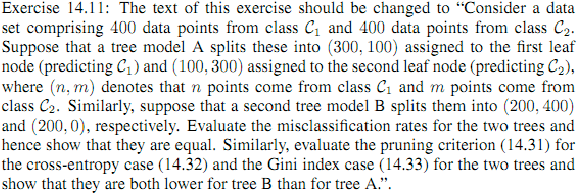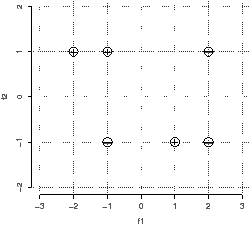# DM825 - Introduction to Machine Learning Sheet 10, Spring 2011 [pdf format]

Exercises 1.29, 1.30, 14.3, 14.1, 14.6, 14.11 of [B1].

Check exercise 14.11. Here is the vresion from the Errata Corrige:## 1  Exercise 1 – Tree based methods

You are given the following data points: Negative: (-1, -1) (2, 1) (2, -1); Positive: (-2, 1) (-1, 1) (1,-1). The points are depicted in Figure 1.Figure 1: The data points for classification.

1. Construct a decision tree using the greedy recursive bi-partitioning algorithm based on information gain described in class. Use both criteria the Gini index and the entropy. In the search for the split threshold θ discretize the continue scale of the two features and consider only values in {−1.5,0,1.5} for f1 and {0} for f2. Represent graphically the tree constructed and draw the decision boundaries in the Figure 1. Table 1 might be useful for some computations

 x y −(x/y) · log(x/y) x y −(x/y) · log(x/y) 1 2 0.50 1 5 0.46 1 3 0.53 2 5 0.53 2 3 0.39 3 5 0.44 1 4 0.50 4 5 0.26 3 4 0.31
 Table 1: Numerical values for the computation of information gains.

2. Use the tree to predict the outcome for the new point (1,1).

## 2  Exercise 2 – Nearest Neighbor

1. Draw the decision boundaries for 1-Nearest Neighbor on the Figure 1. Make it accurate enough so that it is possible to tell whether the integer-valued coordinate points in the diagram are on the boundary or, if not, which region they are in.
2. What class does 1-NN predict for the new point: (1, 1).
3. What class does 3-NN predict for the new point: (1, 0).